# f(r,y)一2 + 2 ry-v2-4r-8y + 9 For Exercises 1-6, find the critical points of the given func- tion and classify them...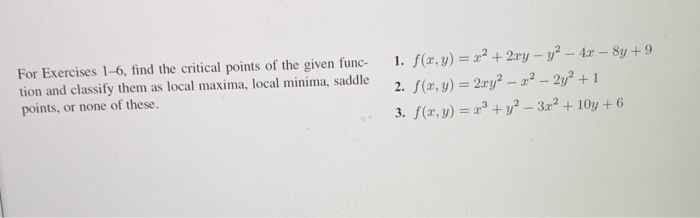f(r,y)一2 + 2 ry-v2-4r-8y + 9 For Exercises 1-6, find the critical points of the given func- tion and classify them as local maxima, local minima, saddle points, or none of these. I. 3. f(z, y) =x3 + y2-3x2 + 10y + 6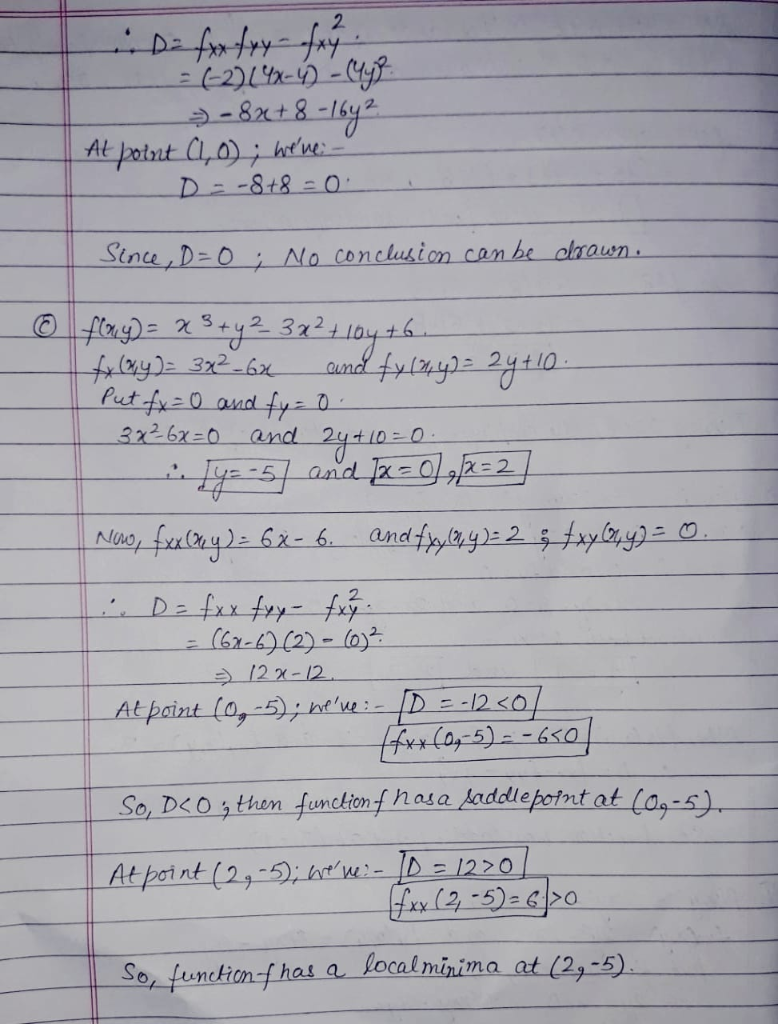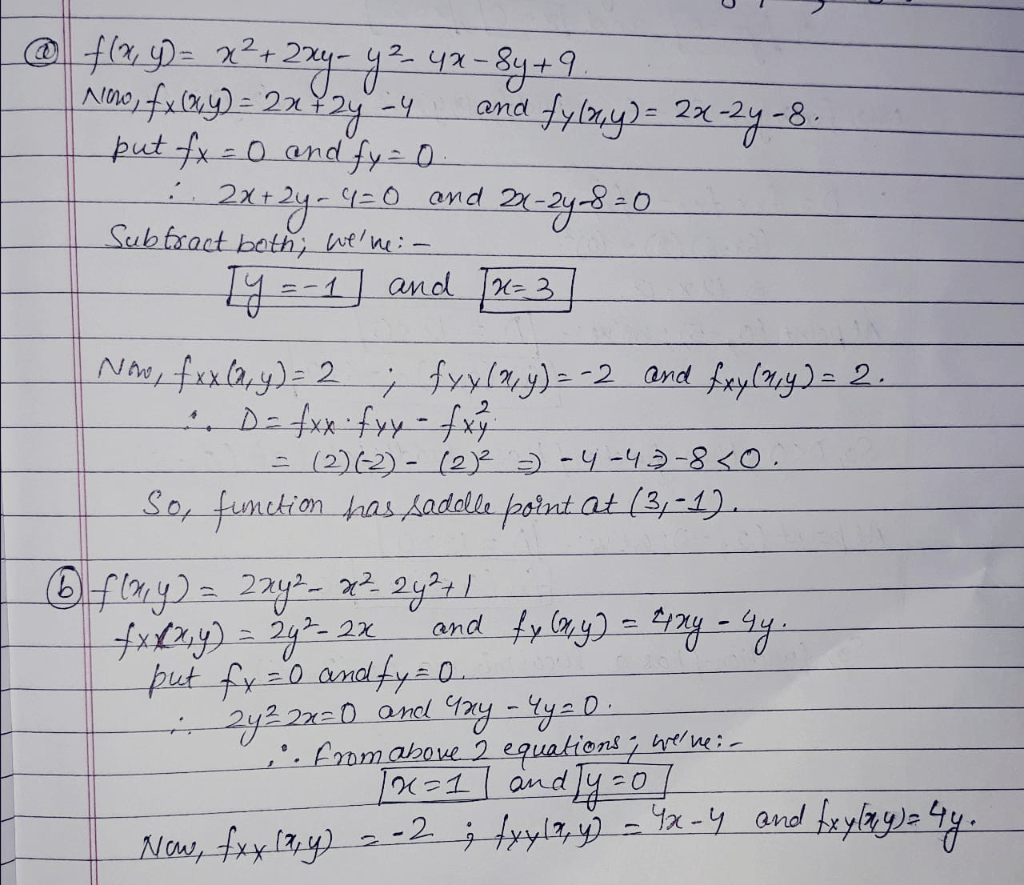##### Add Answer of: f(r,y)一2 + 2 ry-v2-4r-8y + 9 For Exercises 1-6, find the critical points of the given func- tion and classify them...
Similar Homework Help Questions
• ### Let f(x,y)=1+x2−cos(5y). Find all critical points and classify them as local maxima, local minima, saddle points, or non...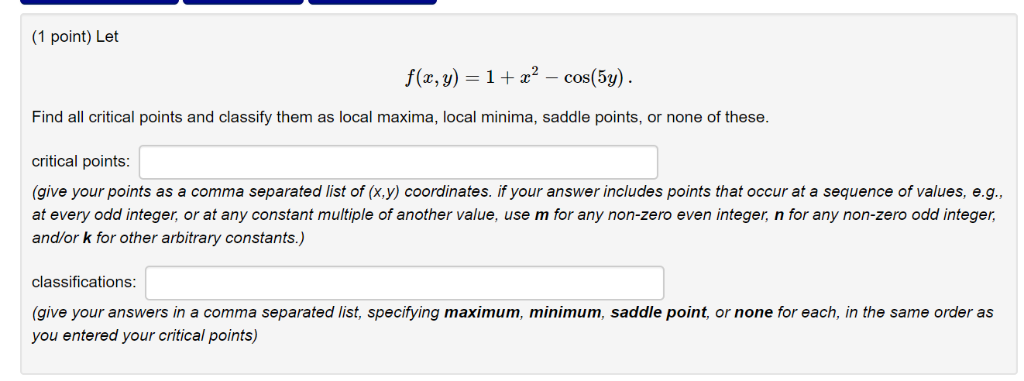Let f(x,y)=1+x2−cos(5y). Find all critical points and classify them as local maxima, local minima, saddle points, or none of these. (1 point) Let Find all critical points and classify them as local maxima, local minima, saddle points, or none of these. critical points (give your points as a comma separated list of (x,y) coordinates. if your answer includes points that occur at a sequence of values, e.g., at every odd integer, or at any constant multiple of another value, use...

• ### 4. (a) (15 points) Find all the local maxima, local minima, and saddle points of the function f(x, y)ry b) (15 points) Use Lagrange multipliers to find the points on the surface ryz-1 closest to the...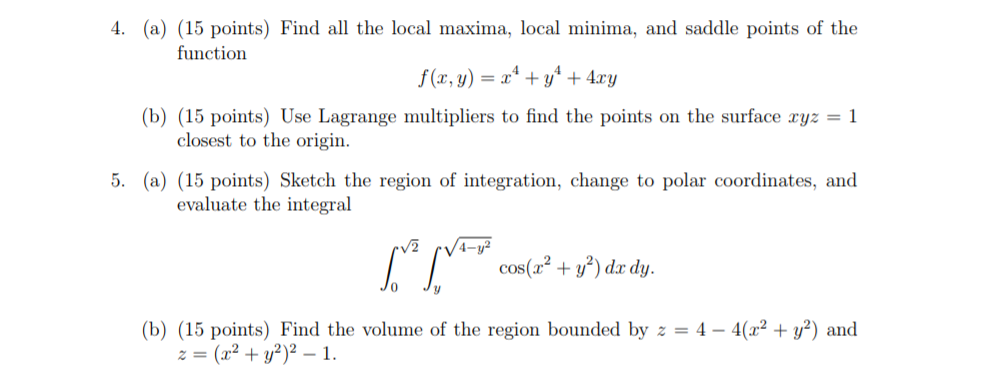4. (a) (15 points) Find all the local maxima, local minima, and saddle points of the function f(x, y)ry b) (15 points) Use Lagrange multipliers to find the points on the surface ryz-1 closest to the origin. polar coordinates, and evaluate the integral cos(a+)d dy. 0 (b) (15 points) Find the volume of the region bounded by z = 4-4(12 + y2) and z=(x2 + y2)2-1. 4. (a) (15 points) Find all the local maxima, local minima, and saddle points...

• ### 2 012 (6 pts). Find the critical points of y and use the second derivative xe 2 1+y2 test to classify these points as saddle, local minimum and local maximum points. 2 012 (6 pts). Find the c...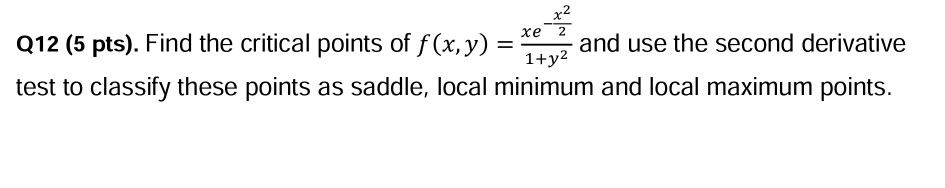2 012 (6 pts). Find the critical points of y and use the second derivative xe 2 1+y2 test to classify these points as saddle, local minimum and local maximum points. 2 012 (6 pts). Find the critical points of y and use the second derivative xe 2 1+y2 test to classify these points as saddle, local minimum and local maximum points.

• ### Question 5 (25 points) : Use Lagrange multipliers to find all the critical points of the function f (x, y, z)-22on the surface 2 -4y2 722. You do not need to determine whether they are maxima, minima...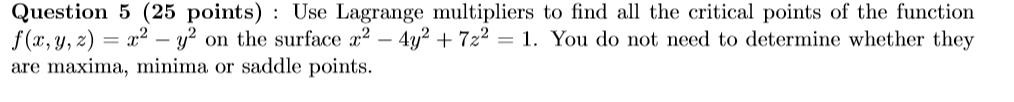Question 5 (25 points) : Use Lagrange multipliers to find all the critical points of the function f (x, y, z)-22on the surface 2 -4y2 722. You do not need to determine whether they are maxima, minima or saddle points. Question 5 (25 points) : Use Lagrange multipliers to find all the critical points of the function f (x, y, z)-22on the surface 2 -4y2 722. You do not need to determine whether they are maxima, minima or saddle points.

• ### (8 points) Find all critical points and classify them via the second derivative test. (a) f(x,y)...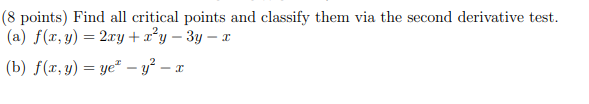(8 points) Find all critical points and classify them via the second derivative test. (a) f(x,y) = 2.ry+y – 3y - 2 (b) f(x,y) = ye" – y? - I

• ### Help! Please do both of them with detailed explanation Find and classify the critical points of z- 28) ( -3y) Loca...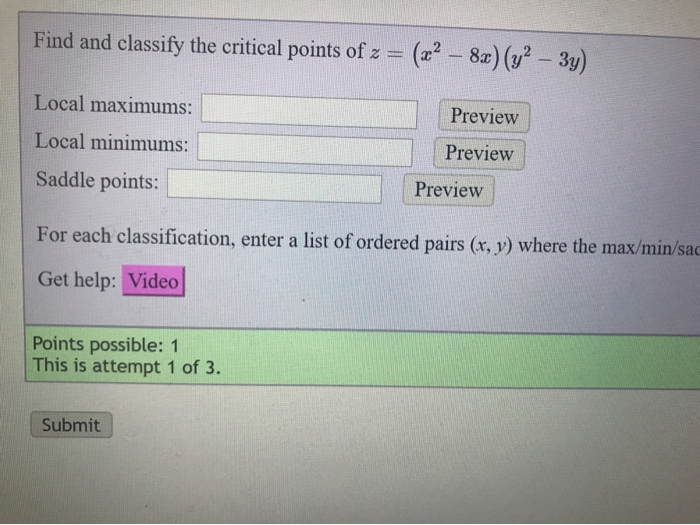Help! Please do both of them with detailed explanation Find and classify the critical points of z- 28) ( -3y) Local maximums: Previevw Local minimums: Preview Saddle points: Preview For each classification, enter a list of ordered pairs (r, y) where the max/min/sac Get help: Video Points possible: 1 This is attempt 1 of 3. Submit Due in 9 Suppose that f(z, y) yy3 3y with D (, y) | 0 y 3) 1. The critical point of f(z, y)...

• ### C) Find the absolute maxima and minima of the function f (x, y) = xy-y^2 over the region -2 < x < 2, -5 < y < 5. on the square -2Srs 2,-5US5 1. (10 points) For the function fa.)y- (a) (4 p...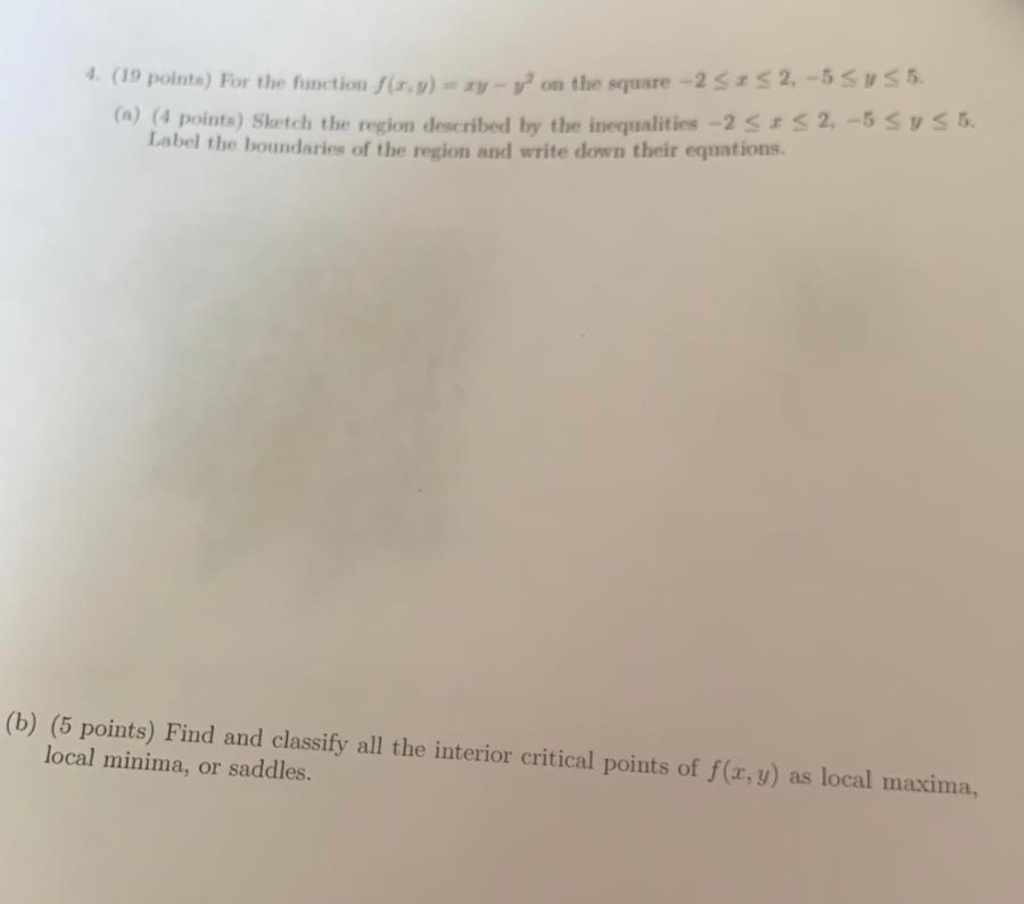C) Find the absolute maxima and minima of the function f (x, y) = xy-y^2 over the region -2 < x < 2, -5 < y < 5. on the square -2Srs 2,-5US5 1. (10 points) For the function fa.)y- (a) (4 points) Shotch the region described by the inequalities -ss2,-5 svs5 Label the boundaries of the region and write down their equations (b) (5 points) Find and classify all the interior critical points of fe, y) as local maxima...

• ### (a) Find and classify all of the critical points of the function X f(x, y, z)...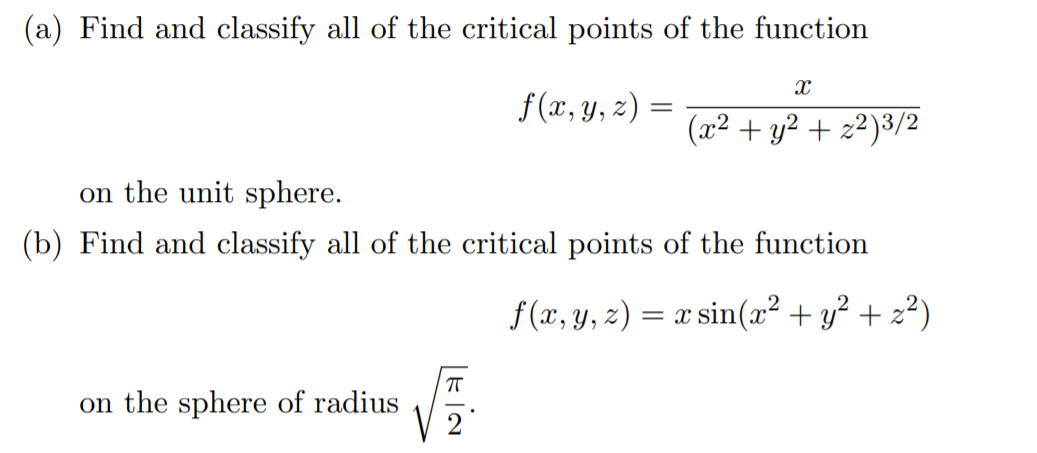(a) Find and classify all of the critical points of the function X f(x, y, z) = (x2 +42 + x2)3/2 on the unit sphere. (b) Find and classify all of the critical points of the function f(x, y, z) = x sin(x2 + y2 +22) on the sphere of radius

• ### (17) Consider the function f that is given by f(x, y)-2y +e Find all its critical points and classify each one as a local maximum, local minimum, or saddle point (17) Consider the function f tha...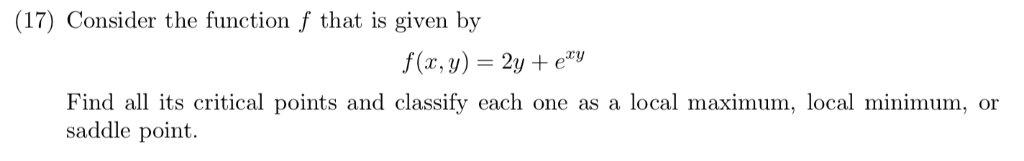(17) Consider the function f that is given by f(x, y)-2y +e Find all its critical points and classify each one as a local maximum, local minimum, or saddle point (17) Consider the function f that is given by f(x, y)-2y +e Find all its critical points and classify each one as a local maximum, local minimum, or saddle point

• ### 6. Find the global maxima and global minima for on the disc r2+y 1. We will divide this into the ...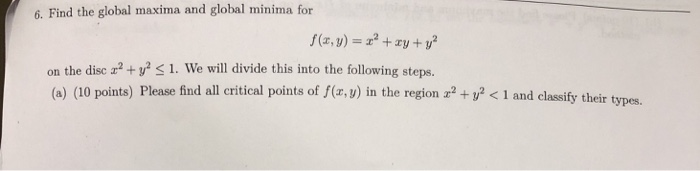6. Find the global maxima and global minima for on the disc r2+y 1. We will divide this into the following steps. (a) (10 points) Please find all critical points of f(r,v) in the region x2 + y2く1 and classify their types. parametrization (b) (10 points) Please find the maximum and minimum of f(r, y) on the boundary z2+y 1 by parametri and minimum of f(r,. y ) on the boundary 2+y2 method. (c) (10 points) Please find the maximum...

Free Homework App